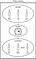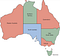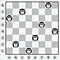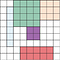# Constraint-Satisfaction Problems in Python

From Classic Computer Science Problems in Python by David Kopec

___________________________________________________________________

Take 42% off Classic Computer Science Problems in Python. Just enter fcckopec2 at checkout at manning.com.
___________________________________________________________________

A large number of problems which computational tools solve can be broadly categorized as constraint-satisfaction problems (CSPs). CSPs are composed of variables with possible values which fall into ranges known as domains. Constraints between the variables must be satisfied in order for constraint-satisfaction problems to be solved. Those three core concepts — variables, domains, and constraints — are simple to understand, and their generality underlies the wide applicability of constraint-satisfaction problem solving.

Let’s consider an example. Suppose you’re trying to schedule a Friday meeting for Joe, Mary, and Sue. Sue has to be at the meeting with at least one other person. For this scheduling problem, the three people — Joe, Mary, and Sue — are the variables. The domain for each variable may be their respective hours of availability. For instance, the variable `Mary` has the domain 2 P.M., 3 P.M., and 4 P.M. This problem also has two constraints. One constraint is that Sue must be at the meeting. The other is that at least two people must attend the meeting. A constraint-satisfaction problem solver is provided with the three variables, three domains, and two constraints, and it solves the problem without requiring that the user explain how. Figure 1 illustrates this example.Figure 1 Scheduling problems are a classic application of constraint-satisfaction frameworks.

Programming languages like Prolog and Picat have built-in facilities for solving constraint-satisfaction problems. The usual technique in other languages is to build a framework that incorporates a backtracking search and several heuristics to improve the performance of that search. In this article, we’ll first build a framework for CSPs that solves them using a simple recursive backtracking search. Then we’ll use the framework to solve several different example problems.

Constraints are defined using a `Constraint` class. Each `Constraint` consists of the `variables` it constrains and a method that checks whether it’s `satisfied()`. The determination of whether a constraint is satisfied is the main logic that goes into defining a specific constraint-satisfaction problem. The default implementation must be overridden because we’re defining our `Constraint` class as an abstract base class. Abstract base classes aren’t meant to be instantiated; only the subclasses that override and implement their `@abstractmethod`s are used.

NOTE

The code in this article makes extensive use of type hints as existent in Python 3.7. If you haven’t seen type hints before checkout the official Python documentation at https://docs.python.org/3/library/typing.html. In addition, there is a type hints kick start in appendix C of Classic Computer Science Problems in Python. Finally, you can find all of the source code from this article online at https://github.com/davecom/ClassicComputerScienceProblemsInPython/tree/master/Chapter3

Listing 1 `csp.py`

`from typing import Generic, TypeVar, Dict, List, Optionalfrom abc import ABC, abstractmethod  V = TypeVar('V') # variable typeD = TypeVar('D') # domain type    # Base class for all constraintsclass Constraint(Generic[V, D], ABC):    # The variables that the constraint is between    def __init__(self, variables: List[V]) -> None:        self.variables = variables      # Must be overridden by subclasses    @abstractmethod    def satisfied(self, assignment: Dict[V, D]) -> bool:        ...`

TIP

Abstract base classes serve as templates for a class hierarchy. They’re more prevalent in other languages, like C++, as a user-facing feature than in Python. In fact, they were only introduced to Python about half-way through the language’s lifetime. With that said, many of the collection classes in Python’s standard library are implemented via abstract base classes. The general advice is not to use them in your own code unless you’re sure that you’re building a framework upon which others will build, and not only an internal use class hierarchy. For more information see Chapter 11 of Fluent Python by Luciano Ramalho.

The centerpiece of our constraint-satisfaction framework is a class called `CSP`. `CSP` is the gathering point for variables, domains, and constraints. In terms of its type hints, it uses generics to make itself flexible enough to work with any kind of variables and domain values (`V` keys and `D` domain values). Within `CSP`, the definitions of the collections `variables`, `domains`, and `constraints` are of types that you’d expect. The `variables` collection is a `list` of variables, `domains` is a `dict` mapping variables to lists of possible values (the domains of those variables), and `constraints` is a `dict` that maps each variable to a `list` of the constraints imposed on it.

Listing 2 `csp.py` continued

`# A constraint satisfaction problem consists of variables of type V# that have ranges of values known as domains of type D and constraints# that determine whether a particular variable's domain selection is validclass CSP(Generic[V, D]):    def __init__(self, variables: List[V], domains: Dict[V, List[D]]) -> None:        self.variables: List[V] = variables # variables to be constrained        self.domains: Dict[V, List[D]] = domains # domain of each variable        self.constraints: Dict[V, List[Constraint[V, D]]] = {}        for variable in self.variables:            self.constraints[variable] = []            if variable not in self.domains:                raise LookupError("Every variable should have a domain assigned to it.")      def add_constraint(self, constraint: Constraint[V, D]) -> None:        for variable in constraint.variables:            if variable not in self.variables:                raise LookupError("Variable in constraint not in CSP")            else:                self.constraints[variable].append(constraint)`

The `__init__()` initializer creates the `constraints dict`. The `add_constraint()` method goes through all of the variables touched by a given constraint and adds itself to the `constraints` mapping for each of them. Both methods have basic error-checking in place, and raise an exception when a `variable` is missing a domain or a `constraint` is on a nonexistent variable.

How do we know if a given configuration of variables and selected domain values satisfy the constraints? We’ll call such a given configuration an “assignment.” We need a function that checks every constraint for a given variable against an assignment to see if the variable’s value in the assignment works for the constraints. Here we implement a `consistent()` function as a method on `CSP`.

Listing 3 `csp.py` continued

`# Check if the value assignment is consistent by checking all constraints# for the given variable against itdef consistent(self, variable: V, assignment: Dict[V, D]) -> bool:    for constraint in self.constraints[variable]:        if not constraint.satisfied(assignment):            return False    return True`

`consistent()` goes through every constraint for a given variable (it’s always a variable that was newly added to the assignment) and checks if the constraint is satisfied, given the new assignment. If the assignment satisfies every constraint, `True` is returned. If any constraint imposed on the variable isn’t satisfied, `False` is returned.

This constraint-satisfaction framework uses a simple backtracking search to find solutions to problems. Backtracking is the idea that once you hit a wall in your search, you go back to the last known point where you made a decision before the wall, and choose a different path. The backtracking search implemented in the following `backtracking_search()` function is a kind of recursive depth-first search. This function’s added as a method to the `CSP` class.

Listing 4 csp.py continued

`def backtracking_search(self, assignment: Dict[V, D] = {}) -> Optional[Dict[V, D]]:    # assignment is complete if every variable is assigned (our base case)    if len(assignment) == len(self.variables):        return assignment      # get all variables in the CSP but not in the assignment    unassigned: List[V] = [v for v in self.variables if v not in assignment]      # get the every possible domain value of the first unassigned variable    first: V = unassigned    for value in self.domains[first]:        local_assignment = assignment.copy()        local_assignment[first] = value        # if we're still consistent, we recurse (continue)        if self.consistent(first, local_assignment):            result: Optional[Dict[V, D]] = self.backtracking_search(local_assignment)            # if we didn't find the result, we will end up backtracking            if result is not None:                return result    return None`

Let’s walk through `backtrackingSearch()`, line by line.

`if len(assignment) == len(self.variables):                   return assignment`

The base case for the recursive search is finding a valid assignment for every variable. Once we have, we return the first instance of a solution that was valid (we stop searching).

`unassigned: List[V] = [v for v in self.variables if v not in assignment]      first: V = unassigned`

To select a new variable whose domain we can explore, we go through all of the variables and find the first that doesn’t have an assignment. To do this, we create a `list` of variables in `self.variables` but not in `assignment` through a list comprehension, and call it `unassigned`. Then we pull out the first value in `unassigned`.

`for value in self.domains[first]:          local_assignment = assignment.copy()          local_assignment[first] = value`

We try assigning every possible domain value for that variable, one at a time. The new assignment for each is stored in a local dictionary called `local_assignment`.

`if self.consistent(first, local_assignment):          result: Optional[Dict[V, D]] = self.backtracking_search(local_assignment)          if result is not None:                  return result`

If the new assignment in `local_assignment` is consistent with all of the constraints (which is what `consistent()` checks for), we continue recursively searching with the new assignment in place. If the new assignment turns out to be complete (the base case), we return the new assignment up the recursion chain.

`return None  # no solution`

Finally, if we’ve gone through every possible domain value for a particular variable, and there’s no solution utilizing the existing set of assignments, we return `None`, indicating no solution. This leads to backtracking up the recursion chain to the point where a different prior assignment could have been made.

The Australian map-coloring problem

Imagine you have a map of Australia that you want to color by state/territory (which we’ll collectively call “regions”). No two adjacent regions should share a color. Can you color the regions with only three different colors?

The answer is yes. Try it out on your own (the easiest way is to print out a map of Australia with a white background). As human beings, we can quickly figure out the solution by inspection and a little trial and error. It’s a trivial problem and a great first problem for our backtracking constraint-satisfaction solver. The problem is illustrated in figure 2.Figure 2 In a solution to the Australian map-coloring problem, no two adjacent parts of Australia can be colored with the same color.

To model the problem as a CSP, we need to define the variables, domains, and constraints. The variables are the seven regions of Australia (at least the seven that we’ll restrict ourselves to): Western Australia; Northern Territory; South Australia; Queensland; New South Wales; Victoria; and Tasmania. In our CSP, they can be modeled with strings. The domain of each variable is the three different colors that can possibly be assigned (we’ll use red, green, and blue). The constraints are the tricky part. No two adjacent regions can be colored with the same color, and our constraints are dependent on which regions border one another. We can use binary constraints (constraints between two variables). Every two regions that share a border also share a binary constraint indicating they can’t be assigned the same color.

To implement these binary constraints in code, we need to subclass the `Constraint` class. The `MapColoringConstraint` subclass takes two variables in its constructor (therefore being a binary constraint): the two regions that share a border. Its overridden `satisfied()` method check whether the two regions both have a domain value (color) assigned to them—if either doesn’t, the constraint’s trivially satisfied until they do (there can’t be a conflict when one doesn’t yet have a color). Then it checks whether the two regions are assigned the same color (obviously there’s a conflict, meaning the constraint isn’t satisfied, when they’re the same).

The class is presented here in its entirety. `MapColoringConstraint` isn’t generic in terms of type hinting, but it subclasses a parameterized version of the generic class `Constraint` that indicates both variables and domains are of type `str`.

Listing 5 map_coloring.py

`from csp import Constraint, CSPfrom typing import Dict, List, Optional    class MapColoringConstraint(Constraint[str, str]):    def __init__(self, place1: str, place2: str) -> None:        super().__init__([place1, place2])        self.place1: str = place1        self.place2: str = place2      def satisfied(self, assignment: Dict[str, str]) -> bool:        # If either place is not in the assignment then it is not        # yet possible for their colors to be conflicting        if self.place1 not in assignment or self.place2 not in assignment:            return True        # check the color assigned to place1 is not the same as the        # color assigned to place2        return assignment[self.place1] != assignment[self.place2]`

TIP

`super()` is sometimes used to call a method on the super class, but one can also use the name of the class itself, as in `Constraint.__init__([place1, place2])`. This is helpful when dealing with multiple inheritance, to know which super class’s method you’re calling.

Now that we have a way of implementing the constraints between regions, fleshing out the Australian map-coloring problem with our CSP solver is a matter of filling in domains and variables, and then adding constraints.

Listing 6 map_coloring.py continued

`if __name__ == "__main__":    variables: List[str] = ["Western Australia", "Northern Territory", "South Australia",                             "Queensland", "New South Wales", "Victoria", "Tasmania"]    domains: Dict[str, List[str]] = {}    for variable in variables:        domains[variable] = ["red", "green", "blue"]    csp: CSP[str, str] = CSP(variables, domains)    csp.add_constraint(MapColoringConstraint("Western Australia", "Northern Territory"))    csp.add_constraint(MapColoringConstraint("Western Australia", "South Australia"))    csp.add_constraint(MapColoringConstraint("South Australia", "Northern Territory"))    csp.add_constraint(MapColoringConstraint("Queensland", "Northern Territory"))    csp.add_constraint(MapColoringConstraint("Queensland", "South Australia"))    csp.add_constraint(MapColoringConstraint("Queensland", "New South Wales"))    csp.add_constraint(MapColoringConstraint("New South Wales", "South Australia"))    csp.add_constraint(MapColoringConstraint("Victoria", "South Australia"))    csp.add_constraint(MapColoringConstraint("Victoria", "New South Wales"))    csp.add_constraint(MapColoringConstraint("Victoria", "Tasmania"))`

Finally, `backtracking_search()` is called to find a solution.

Listing 7 map_coloring.py continued

`solution: Optional[Dict[str, str]] = csp.backtracking_search()  if solution is None:          print("No solution found!")  else:             print(solution)`

A correct solution includes an assigned color for every region.

`{'Western Australia': 'red', 'Northern Territory': 'green', 'South Australia': 'blue', 'Queensland': 'red', 'New South Wales': 'green', 'Victoria': 'red', 'Tasmania': 'green'}`

A chessboard is an eight-by-eight grid of squares. A queen is a chess piece that can move on the chessboard any number of squares along any row, column, or diagonal. A queen is attacking another piece if, in a single move, it can move to the square the piece is on without jumping over any other piece. If the other piece is in the line of sight of the queen, then it’s attacked by it). The eight queens problem poses the question of how eight queens can be placed on a chessboard without any queen attacking another queen. The problem is illustrated in figure 3.Figure 3 In a solution to the eight queens problem (there are many solutions); no two queens can be threatening one another.

To represent squares on the chess board, we’ll assign each an integer row and an integer column. We can ensure each of the eight queens isn’t on the same column by assigning them sequentially the columns 1 through 8. The variables in our constraint-satisfaction problem is the column of the queen in question. The domains can be the possible rows (again 1 through 8). In the following code listing we jump to the end of our file where we define these variables and domains.

`if __name__ == "__main__":          columns: List[int] = [1, 2, 3, 4, 5, 6, 7, 8]          rows: Dict[int, List[int]] = {}          for column in columns:                  rows[column] = [1, 2, 3, 4, 5, 6, 7, 8]          csp: CSP[int, int] = CSP(columns, rows)`

To solve the problem, we need a constraint that checks whether any two queens are on the same row or diagonal (they were all assigned different sequential columns to begin with). Checking for the same row is trivial, but checking for the same diagonal requires a little bit of math. If any two queens are on the same diagonal, the difference between their rows is the same as the difference between their columns. Can you see where these checks take place in `QueensConstraint`? Note that we’re now heading back to the top of our source file to enter the following code.

Listing 9 queens.py continued

`from csp import Constraint, CSPfrom typing import Dict, List, Optional    class QueensConstraint(Constraint[int, int]):    def __init__(self, columns: List[int]) -> None:        super().__init__(columns)        self.columns: List[int] = columns      def satisfied(self, assignment: Dict[int, int]) -> bool:        for q1c, q1r in assignment.items(): # q1c = queen 1 column, q1r = queen 1 row            for q2c in range(q1c + 1, len(self.columns) + 1): # q2c = queen 2 column                if q2c in assignment:                    q2r: int = assignment[q2c] # q2r = queen 2 row                    if q1r == q2r: # same row?                        return False                    if abs(q1r - q2r) == abs(q1c - q2c): # same diagonal?                        return False        return True # no conflict`

Add the constraint and run the search, and we’re back at the bottom of the file.

Listing 10 queens.py continued

`csp.add_constraint(QueensConstraint(columns))  solution: Optional[Dict[int, int]] = csp.backtracking_search()  if solution is None:            print("No solution found!")  else:          print(solution)`

Notice that we’re able to reuse the constraint-satisfaction problem-solving framework which we built for map coloring for a completely different type of problem. This is the power of writing code generically! Algorithms should be implemented in as broadly applicable a manner as possible, unless a performance optimization for a particular application requires specialization.

A correct solution assigns a column and row to every queen.

`{1: 1, 2: 5, 3: 8, 4: 6, 5: 3, 6: 7, 7: 2, 8: 4}`

SEND+MORE=MONEY is a cryptarithmetic puzzle, meaning it’s about finding digits that replace letters to make a mathematical statement true. Each letter in the problem represents one digit (0–9). No two letters can represent the same digit. When a letter repeats, it means a digit repeats in the solution.

To solve this puzzle by hand, it helps to line up the words.

` SEND   +MORE  =MONEY`

It’s solvable by hand, with a bit of algebra and intuition, but a simple computer program can solve it faster by brute forcing many possible solutions. Let’s represent SEND+MORE=MONEY as a constraint-satisfaction problem.

Listing 11 send_more_money.py

`from csp import Constraint, CSPfrom typing import Dict, List, Optional    class SendMoreMoneyConstraint(Constraint[str, int]):    def __init__(self, letters: List[str]) -> None:        super().__init__(letters)        self.letters: List[str] = letters      def satisfied(self, assignment: Dict[str, int]) -> bool:        # if there are duplicate values then it's not a solution        if len(set(assignment.values())) < len(assignment):            return False          # if all variables have been assigned, check if it adds correctly        if len(assignment) == len(self.letters):            s: int = assignment["S"]            e: int = assignment["E"]            n: int = assignment["N"]            d: int = assignment["D"]            m: int = assignment["M"]            o: int = assignment["O"]            r: int = assignment["R"]            y: int = assignment["Y"]            send: int = s * 1000 + e * 100 + n * 10 + d            more: int = m * 1000 + o * 100 + r * 10 + e            money: int = m * 10000 + o * 1000 + n * 100 + e * 10 + y            return send + more == money        return True # no conflict`

`SendMoreMoneyConstraint`’s `satisfied()` method does a few things. First, it checks if there are any letters representing the same digits. If there are, it’s an invalid solution, and it returns `False`. Next, it checks if all letters have been assigned. If they have, it checks to see if the formula (SEND+MORE=MONEY) is correct with the given assignment. If it is, a solution has been found, and it returns `True`. Otherwise, it returns `False`. Finally, if all letters haven’t yet been assigned, it returns `True`. This is to ensure that a partial solution continues to be worked on.

Let’s try running it:

Listing 12 send_more_money.py continued

`if __name__ == "__main__":    letters: List[str] = ["S", "E", "N", "D", "M", "O", "R", "Y"]    possible_digits: Dict[str, List[int]] = {}    for letter in letters:        possible_digits[letter] = [0, 1, 2, 3, 4, 5, 6, 7, 8, 9]    possible_digits["M"] =   # so we don't get answers starting with a 0    csp: CSP[str, int] = CSP(letters, possible_digits)    csp.add_constraint(SendMoreMoneyConstraint(letters))    solution: Optional[Dict[str, int]] = csp.backtracking_search()    if solution is None:        print("No solution found!")    else:        print(solution)`

Note that we preassigned the answer for the letter M. This was to ensure that the answer doesn’t include a zero for M, because our constraint has no notion of the concept that a number can’t start with zero. Feel free to try it out without that preassigned answer.

The solution should look something like this:

`{'S': 9, 'E': 5, 'N': 6, 'D': 7, 'M': 1, 'O': 0, 'R': 8, 'Y': 2}`

A manufacturer needs to fit certain rectangular chips onto a rectangular circuit board. This problem asks, “how can several different-sized rectangles all fit snugly inside of another rectangle?” A constraint-satisfaction problem solver can find the solution. The problem is illustrated in figure 4.Figure 4 The circuit board layout problem is similar to a word-search problem, but the rectangles are of variable width.

As was mentioned in the introduction to this article, constraint-satisfaction problem solvers are commonly used in scheduling. Several people need to be at a meeting, and they are the variables. The domains consist of the open times on their calendars. The constraints may involve what combinations of people are required at the meeting.

Constraint-satisfaction problem solvers are also used in motion planning. Imagine a robot arm that needs to fit inside of a tube. It has constraints (the walls of the tube), variables (the joints), and domains (possible movements of the joints).

Applications in computational biology are numerous. You can imagine constraints between molecules required for a chemical reaction. And, as is common with AI, there are applications in games. Writing a Sudoku solver is one of the following exercises, but many logic puzzles can be solved using constraint-satisfaction problem solving.

1. Build the circuit board layout problem solver as described in the article, if you haven’t already.
2. Build a program that can solve Sudoku problems using this article’s constraint-satisfaction problem framework.